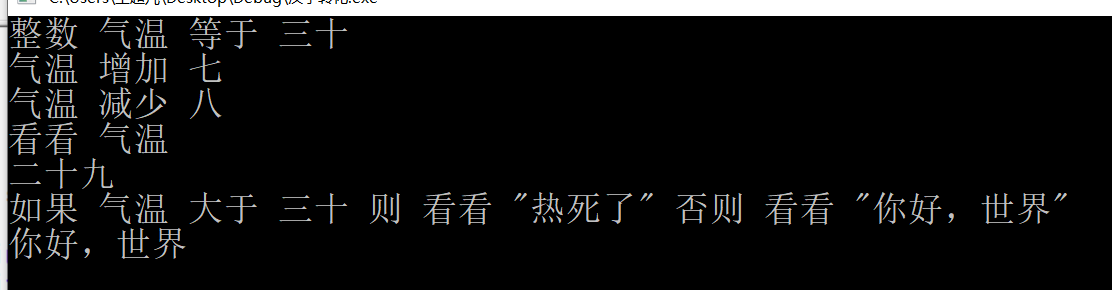# 编程要求

### 请编写一个程序，制作一个简易的中文语言编译器，即使用中文语法进行编程，输入为逐行输入，每行为一个语句，一个语句代变一个操作，满足以下语法要求（括号内代变格式类型，具体参考样例）：

• 变量定义：整数 （变量名） 等于 （数字）
• 运算（加法）：（变量名） 增加 （数字）
• 运算（减法）：（变量名） 减少 （数字）
• 输出：看看 （变量名） or 看看 “（字符串内容）”

### 附加要求：

• 选择：如果 （判断语句） 则 （操作语句） 否则 （操作语句）
• 若否则后没有任何操作使用（无）来进行填充（参考样例2）。

### 注意事项:

• 输入输出采用utf-8编码，请在编码过程中注意。
• 数字只会出现：零一二三四五六七八九十。(此条作废，可先完成0-10的情况)
• 字符串输出的双引号是全角，即中文双引号。
• 变量类型要求只有整数，如有余力，可以自己实现更多。
• 测试数据会相较于样例复杂，请考虑充分需求。
• 不限制编程语言。
• 不能使用eval函数动态执行代码，或调用系统指令。
• 数据输入形式为交互式运行，类似于shell。

### 分析：

• 汉字和数字之间的转换
• 变量的判断
• 对“如果”与“看看”之间的判断
• 去除引号的字符串输出

### 代码分解：

• 将汉字转换成数字：
int hz(char a[]){//进行汉字->数字转变
if (strcmp(a,"零") == 0) return 0;
if (strcmp(a,"一") == 0) return 1;
if (strcmp(a,"二") == 0) return 2;
if (strcmp(a,"三") == 0) return 3;
if (strcmp(a,"四") == 0) return 4;
if (strcmp(a,"五") == 0) return 5;
if (strcmp(a,"六") == 0) return 6;
if (strcmp(a,"七") == 0) return 7;
if (strcmp(a,"八") == 0) return 8;
if (strcmp(a,"九") == 0) return 9;
if (strcmp(a,"十") == 0) return 10;
}

int hanzi(char sum[])//将汉字变成数字
{
char a,b;
if(strlen(sum)==2){
return	hz(sum);//零到十的数字
}
else if(strlen(sum)==4){
a=sum;//将十位存放在数组a中
a=sum;
a='\0';
b=sum;//将个位存放在数组b中
b=sum;
b='\0';
if(hanzi(a)==10)
return hz(b)+10;//十到二十之间的数字,十一，十二
else
return 10*hz(a);//二位整数（如：20,30）
}
else if(strlen(sum)==6){
a=sum;//将个位存放在数组a中
a=sum;
a='\0';
b=sum;//将十位位存放在数组b中
b=sum;
b='\0';
return hz(a)+hz(b)*10;//二十以上，九十九以下的数字,例如三十二
}
}


• 将数字转换成汉字
void shuzi(int sum)//将数字变成汉字
{
int a,b;
if(sum<20)
sz(sum);
else{
a=sum/10;//等于十位数
sz(a);
printf("十");
if(sum%10!=0){//判断是不是整数
b=sum%10;//等于个位数
sz(b);
}
}
}

void sz(int sum){//进行数字->汉字转变
if(sum<=10){
if(sum==0) printf("零");
else if(sum==1) printf("一");
else if(sum==2) printf("二");
else if(sum==3) printf("三");
else if(sum==4) printf("四");
else if(sum==5) printf("五");
else if(sum==6) printf("六");
else if(sum==7) printf("七");
else if(sum==8) printf("八");
else if(sum==9) printf("九");
else if(sum==10) printf("十");
}
if(sum>10 && sum <20){
if(sum==11) printf("十一");
else if(sum==12) printf("十二");
else if(sum==13) printf("十三");
else if(sum==14) printf("十四");
else if(sum==15) printf("十五");
else if(sum==16) printf("十六");
else if(sum==17) printf("十七");
else if(sum==18) printf("十八");
else if(sum==19) printf("十九");
}
}


• 主函数：
int main()
{
int sum, temp,i;
char a, b,c,d,e,f; //a用来暂存汉字,b存储变量
scanf("%s",a);
scanf("%s",b);//用于储存代表变量的汉字，如钱包，银行卡之类的
//printf("%s",b);
if (strcmp(a,"等于") != 0){
scanf("%s",a);//判断是否是“等于”,是退出判断，不是继续
}
scanf("%s",a);//赋初值
sum = hanzi(a);//将赋初值用hanzi函数用于汉字转为数字
while(scanf("%s",a)!=EOF){
if(strcmp(a,b)==0){
scanf("%s",c);
if(strcmp(c,"增加")==0){
scanf("%s",c);
temp=hanzi(c);
sum=sum+temp;
}
if(strcmp(c,"减少")==0){
scanf("%s",c);
temp=hanzi(c);
sum=sum-temp;
}
if(sum<0 || sum>99)
printf("超出范围");
}
else if(strcmp(a,"如果")==0){
scanf("%s",a);
if(strcmp(a,b)==0){
scanf("%s",c);
if(strcmp(c,"大于")==0){
scanf("%s",c);
temp=hanzi(c);
scanf("%s %s %s",c,d,e);
if(sum>temp){
for(i=1;i<strlen(e)-2;i=i+2)
printf("%c%c",e[i],e[i+1]);
printf("\n");
}
else{
scanf("%s %s %s",c,d,e);
for(i=1;i<strlen(e)-2;i=i+2)
printf("%c%c",e[i],e[i+1]);
printf("\n");
}
}
}
else
printf("没有此变量");
}
if(strcmp(a,"看看")==0){
scanf("%s",c);
if(strcmp(c,b)==0)
shuzi(sum);
}
}
return 0;
}


### 测试：### 目前还存在的问题：

1、目前代码还不能支持多个变量问题
2、只考虑到整数0~99之间的数
3、目变化的类型还只是整数

### 心得体会：

突然写代码作业，有一点不适应了，主要是以前学习的语言差不多都忘了，导致编程起来很困难。每次都需要去查阅大量的知识点，比如什么strcmp怎么用，一开始进行转换就是傻傻的一个个去匹配，后来网上看看别人的代码思路，然后结合了自己的理解才写了出来。所以接下来一段时间要把以前的编程语言（至少一门）的基础再次给补回来。
posted @ 2020-02-09 19:07  wang295  阅读(215)  评论(0编辑  收藏